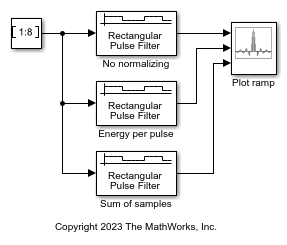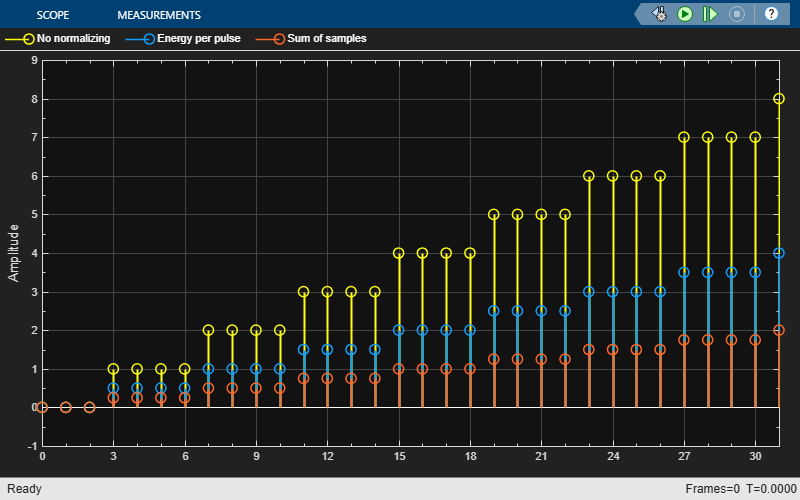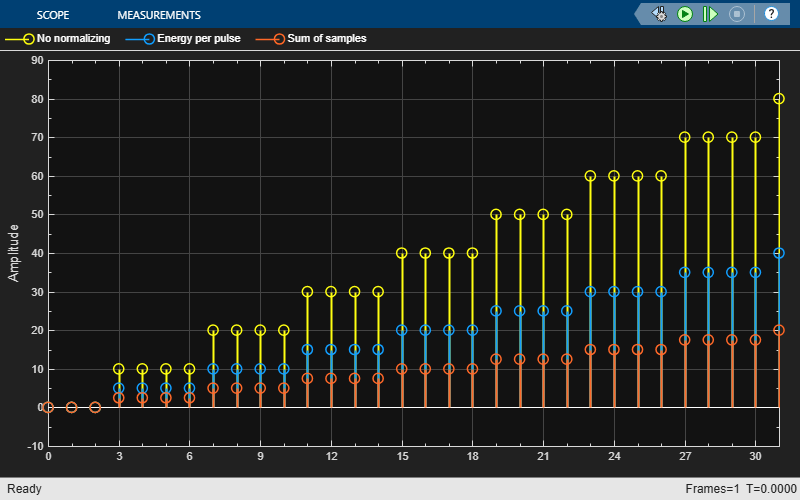# Ideal Rectangular Pulse Filter

Shape input signal using ideal rectangular pulses

•Libraries:
Communications Toolbox / Comm Filters

## Description

The Ideal Rectangular Pulse Filter block upsamples and shapes the input signal using rectangular pulses. After replicating input samples, the block can also normalize the output signal and apply a linear amplitude gain.

## Examples

expand all

Apply ideal rectangular filtering to a ramp signal.

The `cm_ideal_rect_pulse_filter` model plots a ramp signal with various normalization methods applied. The model includes three Ideal Rectangular Pulse Filter blocks configured with Pulse length (number of samples) set to `4` and Pulse delay (number of samples) set to `3` to filter a ramp input signal, and an Array Plot block with default settings to display one frame of the output signals.Run the model with Linear amplitude gain of the filters set to `1`. The first three samples are zero because the filter blocks have pulse delay set to `3`.Run the model with Linear amplitude gain of filters set to `10`.## Ports

### Input

expand all

Input signal, specified as a scalar, column vector, or Ki-by-N matrix. Ki is the number of input samples per signal channel, and N is the number of signal channels.

Data Types: `double` | `single` | `fixed point`

### Output

expand all

Output signal, returned as a scalar, column vector, or matrix. The block filters each channel over time and generates a Ko-by-N output matrix. The output signal is the same data type as the input signal.

• Ko = Ki × L.

• N is the number of signal channels.

• Ki is the number of input samples per signal channel.

• L is the value you specify in the Pulse length (number of samples) parameter.

Data Types: `double` | `single` | `fixed point`

## Parameters

expand all

To edit block parameters interactively, use the Property Inspector. From the Simulink® Toolstrip, on the Simulation tab, in the Prepare gallery, select .

Main

Pulse length in samples, specified as a positive integer. The block replicates each input sample L times, where L is the pulse length that you specify in samples.

Pulse delay in samples, specified as a nonnegative integer. If this parameter is nonzero, then the block outputs that number of zeros at the beginning of the simulation, before starting to replicate any of the input values.

Input processing control, specified as one of these options:

• `Columns as channels (frame based)` — The block treats each column of the input as a separate channel.

• `Elements as channels (sample based)` — The block treats each element of the input as a separate channel.

Block processing rate for input signal upsampling and filtering, specified as one of these options:

• `Enforce single-rate processing` — When you select this option, the block maintains the input sample rate and processes the signal by increasing the output frame size by a factor of N.

• `Allow multirate processing` — When you select this option, the block processes the signal such that the output sample rate is N times faster than the input sample rate.

#### Dependencies

To use `Enforce single-rate processing`, you must set Input processing to ```Columns as channels (frame based)```.

Select this parameter to scale the set of replicated values before applying the linear amplitude gain.

Normalization method, specified as `Sum of samples` or `Energy per pulse`. This parameter assigns the quantity that the block considers when scaling the set of replicated values. For more information, see Normalization Methods.

#### Dependencies

This parameter appears when you select Normalize output signal.

Linear gain, specified as a positive scalar. The block scales the output signal by the linear gain value that you specify. For more information, see Normalization Methods.

Data Types

Select the rounding mode for fixed-point operations. The block uses rounding mode when the result of a fixed-point calculation does not map exactly to a number representable by the data type. The filter coefficients do not obey this parameter; they always round to `Nearest`. For more information, see Rounding Modes or Rounding Mode: Simplest (Fixed-Point Designer).

Select the overflow mode for fixed-point operations. The filter coefficients do not obey this parameter; they are always saturated.

Specify the word length and the fraction length of the filter coefficients (numerator and denominator). For illustrations depicting the use of the coefficient data types in this block, see Filter Structure Diagrams.

When you select `Inherit: Same word length as input`, the word length of the filter coefficients match that of the input to the block. In this mode, the fraction length of the coefficients is automatically set to the binary-point-only scaling that provides you with the best precision possible given the value and word length of the coefficients.

The filter coefficients do not obey the Rounding mode and the Saturate on integer overflow parameters; they are always saturated and rounded to `Nearest`.

For information about specifying data types, see Data Type Assistant.

Specify the product output word and fraction lengths. For diagrams showing the use of the product output data type in this block, see the filter structure diagrams for the Discrete FIR Filter (Simulink) block and Multiplication Data Types.

• When you select ```Inherit: Inherit via internal rule```, the block inherits the data type based on an internal rule. For more information on this rule, see Inherit via Internal Rule.

• When you select ```Inherit: Same as input```, these characteristics match those of the input to the block.

For information about specifying data types, see Data Type Assistant.

Specify the accumulator word and fraction lengths. For diagrams showing the use of the accumulator output data type in this block, see the filter structure diagrams for the Discrete FIR Filter (Simulink) block and Multiplication Data Types.

• When you select ```Inherit via internal rule```, the block inherits the data type based on an internal rule. For more information on this rule, see Inherit via Internal Rule.

• When you select ```Inherit: Same as input```, these characteristics match those of the input to the block.

• When you select ```Inherit: Same as product output```, these characteristics match those of the product output.

For information about specifying data types, see Data Type Assistant.

Specify the output word and fraction lengths.

• When you select ```Inherit: Same as input```, these characteristics match those of the input to the block.

• When you select ```Inherit: Same as accumulator```, these characteristics match those of the accumulator.

• When you select ```Inherit: Same as product output```, these characteristics match those of the product output.

For information about specifying data types, see Data Type Assistant.

Select this parameter to prevent the fixed-point tools from overriding the data types that you specify in the block dialog box. For more information, see the Fixed-Point Tool (Fixed-Point Designer).

## Block Characteristics

 Data Types `double` | `fixed pointa` | `single` Multidimensional Signals `no` Variable-Size Signals `yes` a Fixed-point outputs must be signed.

expand all

expand all

## Version History

Introduced before R2006a